# Titanic Analysis Practice¶

The primary targets of this practice:

1. Take this excercise as a preparation for kaggle competitions
2. Hands-on experience to data analysis, cleaning and modeling
3. Review visual analytic skills (matplotlib and seaborn)
4. Use interactive ploting module: plotly (taking a Udemy course now)

Let's do it!

In :
import pandas as pd
import matplotlib.pyplot as plt
import seaborn as sns
import numpy as np

# filter warnings
import warnings
warnings.filterwarnings('ignore')
from sklearn.exceptions import DataConversionWarning
warnings.filterwarnings(action='ignore')

%matplotlib inline
sns.set_style('darkgrid')


## Define the problem¶

The problem is to predict the survivals in titanic.

## Gather the Data¶

As the data is already given in the competition, all we need to do is to read them.

In :
gender_submission = pd.read_csv('titanic/gender_submission.csv')


## Prepare Data¶

In :
print(train_df.info())
train_df.sample(3)

<class 'pandas.core.frame.DataFrame'>
RangeIndex: 891 entries, 0 to 890
Data columns (total 12 columns):
PassengerId    891 non-null int64
Survived       891 non-null int64
Pclass         891 non-null int64
Name           891 non-null object
Sex            891 non-null object
Age            714 non-null float64
SibSp          891 non-null int64
Parch          891 non-null int64
Ticket         891 non-null object
Fare           891 non-null float64
Cabin          204 non-null object
Embarked       889 non-null object
dtypes: float64(2), int64(5), object(5)
memory usage: 83.6+ KB
None

Out:
PassengerId Survived Pclass Name Sex Age SibSp Parch Ticket Fare Cabin Embarked
681 682 1 1 Hassab, Mr. Hammad male 27.0 0 0 PC 17572 76.7292 D49 C
321 322 0 3 Danoff, Mr. Yoto male 27.0 0 0 349219 7.8958 NaN S
68 69 1 3 Andersson, Miss. Erna Alexandra female 17.0 4 2 3101281 7.9250 NaN S
In :
train_df.describe(include='all')

Out:
PassengerId Survived Pclass Name Sex Age SibSp Parch Ticket Fare Cabin Embarked
count 891.000000 891.000000 891.000000 891 891 714.000000 891.000000 891.000000 891 891.000000 204 889
unique NaN NaN NaN 891 2 NaN NaN NaN 681 NaN 147 3
top NaN NaN NaN Stephenson, Mrs. Walter Bertram (Martha Eustis) male NaN NaN NaN 347082 NaN B96 B98 S
freq NaN NaN NaN 1 577 NaN NaN NaN 7 NaN 4 644
mean 446.000000 0.383838 2.308642 NaN NaN 29.699118 0.523008 0.381594 NaN 32.204208 NaN NaN
std 257.353842 0.486592 0.836071 NaN NaN 14.526497 1.102743 0.806057 NaN 49.693429 NaN NaN
min 1.000000 0.000000 1.000000 NaN NaN 0.420000 0.000000 0.000000 NaN 0.000000 NaN NaN
25% 223.500000 0.000000 2.000000 NaN NaN 20.125000 0.000000 0.000000 NaN 7.910400 NaN NaN
50% 446.000000 0.000000 3.000000 NaN NaN 28.000000 0.000000 0.000000 NaN 14.454200 NaN NaN
75% 668.500000 1.000000 3.000000 NaN NaN 38.000000 1.000000 0.000000 NaN 31.000000 NaN NaN
max 891.000000 1.000000 3.000000 NaN NaN 80.000000 8.000000 6.000000 NaN 512.329200 NaN NaN

Understanding the data:

Column Name Data Type Comment
Survived binary nominal it's the training label for our estimator
PassengerId nominal it's a random number which is not helpful in the analysis
Name nominal it shows information like gender and title
Sex nominal need to be converted
Embarked nominal the place people embarked to the ship.need to be converted
Ticket nominal seems not useful
Cabin nominal lot's of missing data
Pclass ordinal as stated in kaggle, it stands for ticket class
Age continuous quantitative datatypes
Fare continuous quantitative datatypes
SibSp discrete quantitative datatypes number of siblings and spouses
Parch discrete quantitative datatypes number of parents and children

## The 4 C's of Data Cleaning: Correcting, Completing, Creating, and Converting¶

### Correcting errors¶

To see if there's any aberrant or non-acceptable data inputs.

In :
# first, we create a copy of the original training dataset
# as we are now preparing for EDA so we call it df_EDA
df_EDA = train_df.copy()

# The ID is just a random number
df_EDA.drop(['PassengerId'],axis=1,inplace=True)


### Completing missing information¶

Check for null values or missing data.

In :
# missing data
df_EDA.isnull().sum()[df_EDA.isnull().sum() != 0]

Out:
Age         177
Cabin       687
Embarked      2
dtype: int64

Age

In :
# stats info
print(df_EDA.Age.describe())

# shape of its distribution
f, axes = plt.subplots(1,2,figsize=(12,4))

sns.boxplot(df_EDA.Age.dropna(),ax=axes)
sns.distplot(df_EDA.Age.dropna(),ax=axes)

count    714.000000
mean      29.699118
std       14.526497
min        0.420000
25%       20.125000
50%       28.000000
75%       38.000000
max       80.000000
Name: Age, dtype: float64

Out:
<matplotlib.axes._subplots.AxesSubplot at 0x13333811240>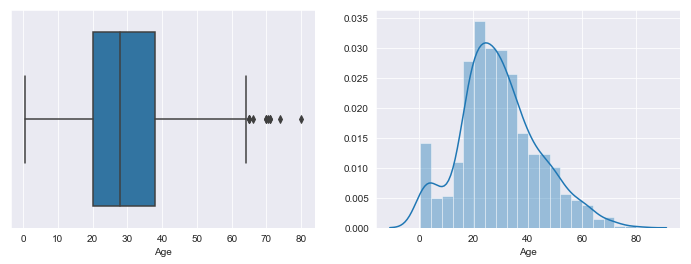From the data and the graphs above, we can know that its a slightly right-skewed normal distribution. Taking the median to fill all the null value should be fine, but we want more accuracy. First, let's see what it's like to fill with median. Wei: expectation maximization.

In :
# fill age with median, save df for comparison
test_df = df_EDA.Age.fillna(df_EDA.Age.median())


What if we look into the feature correlations and fill the median according to different situations?

In :
# convert sex into categories
df_EDA['Sex_Code'] = df_EDA['Sex'].astype('category').cat.codes

# show correlation heatmaps

Out:
Survived Pclass Age SibSp Parch Fare Sex_Code
Survived 1 -0.338481 -0.0772211 -0.0353225 0.0816294 0.257307 -0.543351
Pclass -0.338481 1 -0.369226 0.0830814 0.0184427 -0.5495 0.1319
Age -0.0772211 -0.369226 1 -0.308247 -0.189119 0.0960667 0.0932536
SibSp -0.0353225 0.0830814 -0.308247 1 0.414838 0.159651 -0.114631
Parch 0.0816294 0.0184427 -0.189119 0.414838 1 0.216225 -0.245489
Fare 0.257307 -0.5495 0.0960667 0.159651 0.216225 1 -0.182333
Sex_Code -0.543351 0.1319 0.0932536 -0.114631 -0.245489 -0.182333 1

The most correlated columns for age is "Pclass", "SibSp" and "Parch". I'm going to plot and see if that's intuitively ture.

In :
sns.catplot(data=df_EDA,x='Pclass', y='Age', hue='Sex',
row='SibSp', col='Parch',kind='box',
row_order=[0,1,2],col_order=[0,1,2])

Out:
<seaborn.axisgrid.FacetGrid at 0x13333c31160>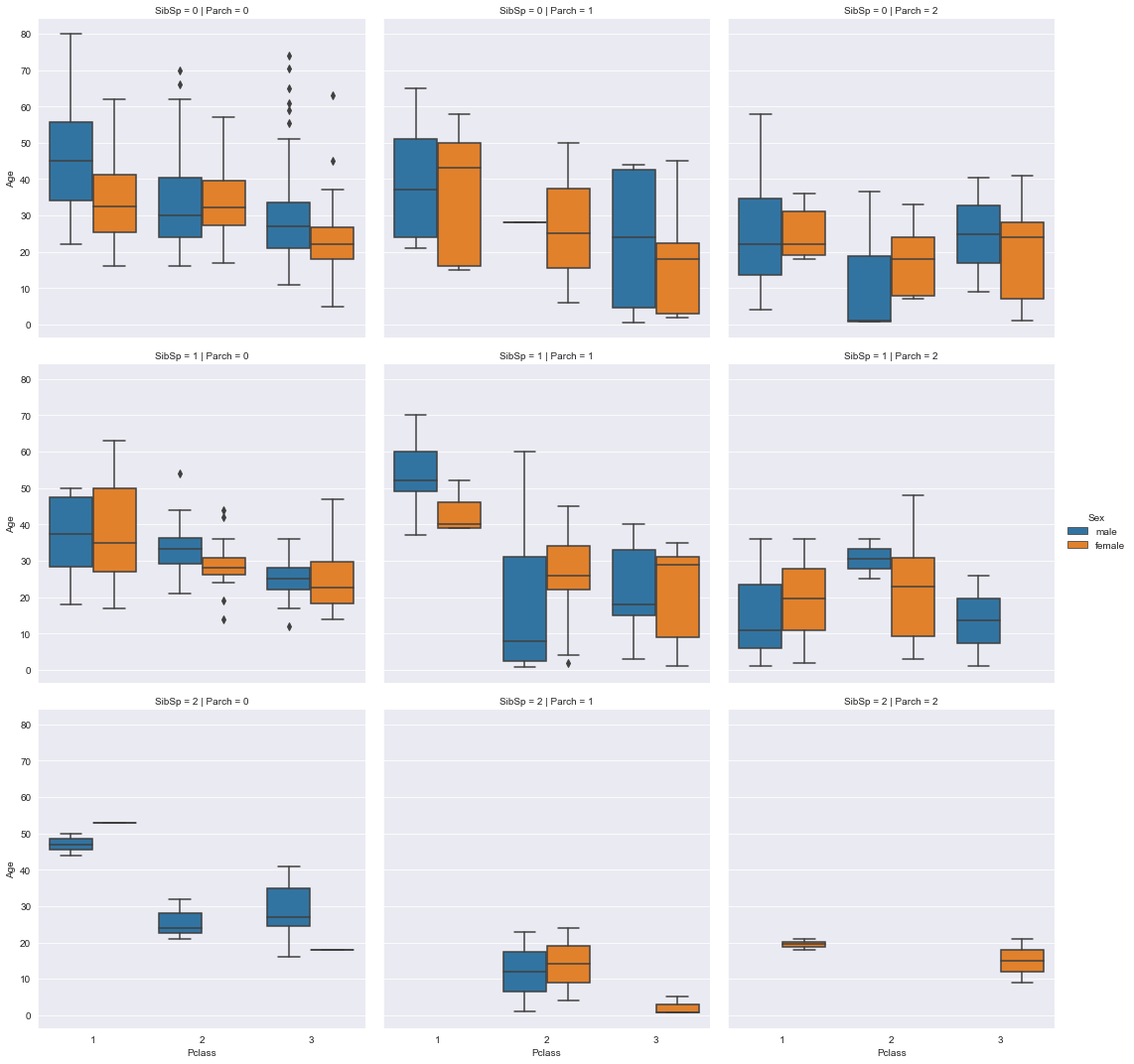We are gonna calculate median age for different sex, pclass, sibch and parch and fill NA with them.

In :
# Fill NA with a complex method
for ind in df_EDA[df_EDA.Age.isnull()].index:
# filters
filter1 = df_EDA['Sex'] == df_EDA.loc[ind, 'Sex']
filter2 = df_EDA['Pclass'] == df_EDA.loc[ind, 'Pclass']
filter3 = df_EDA['SibSp'] == df_EDA.loc[ind, 'SibSp']
filter4 = df_EDA['Parch'] == df_EDA.loc[ind, 'Parch']
fill_value = df_EDA[filter1][filter2][filter3][filter4]['Age'].median()

# if filter result is nan, we fill with the global median
if pd.isna(fill_value):
fill_value = df_EDA['Age'].median()

# fill in values
df_EDA.loc[ind, 'Age'] = fill_value

# stats info
print(df_EDA.Age.describe(),"\n")

print("The kurtosis of the complex method is: {}".format(df_EDA.Age.kurtosis()))
print("The kurtosis of the global median method is: {}".format(test_df.kurtosis()))

# shape of its distribution
f, axes = plt.subplots(2,2,figsize=(12,8))

sns.boxplot(df_EDA.Age.dropna(),ax=axes[0,0])
sns.distplot(df_EDA.Age.dropna(),ax=axes[0,1])
sns.boxplot(test_df,ax=axes[1,0],color='Orange')
sns.distplot(test_df,ax=axes[1,1],color='Orange')

axes[0,0].set_title('Box Plot')
axes[0,1].set_title('Dist Plot')
axes[0,0].set_ylabel('More complex method')
axes[1,0].set_ylabel('Fill with median')

count    891.000000
mean      29.387957
std       13.467945
min        0.420000
25%       22.000000
50%       27.000000
75%       36.000000
max       80.000000
Name: Age, dtype: float64

The kurtosis of the complex method is: 0.5874999132251677
The kurtosis of the global median method is: 0.9938710163801736

Out:
Text(0, 0.5, 'Fill with median')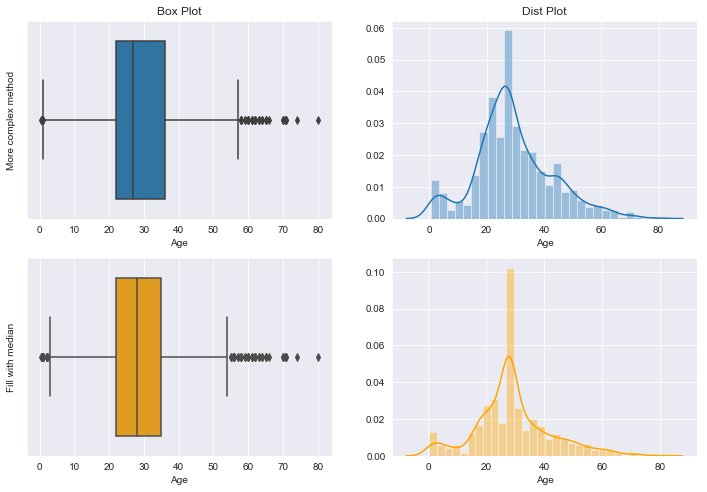It can be clearly seen that the complex method is much better. It create less outliers, reduce the kurtosis and makes more sense intuitively.

Cabin

In :
df_with_cabin = df_EDA[df_EDA.Cabin.notnull()]

# Let us see the survival rate
rate_of_survival_cabin = df_with_cabin.Survived.mean()
print("Survival Rate:","{:.0%}".format(rate_of_survival_cabin))

df_with_cabin['Cabin'] = df_with_cabin.Cabin.apply(lambda x: x)

sns.barplot(x='Cabin',y='Survived',data=df_with_cabin)

Survival Rate: 67%

Out:
<matplotlib.axes._subplots.AxesSubplot at 0x13334c4dac8>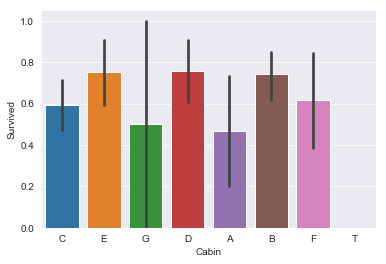In [ ]:
df_EDA['Cabin'] = (df_EDA.Cabin
.apply(lambda x: x if pd.notna(x) else 'Z')
.apply(lambda x: 'Y' if x in ['C','E','D','B','F'] else x)
.apply(lambda x: 'X' if x in ['G','A','T'] else x))

print(a.value_counts())
sns.barplot(x=a, y=train_df.Survived)


A survival rate of 66% is really high comparing to the overall rate! Remember that we have the overall survival rate of 38%.

We already know that ticket fare and pclass are the most important factor, so I guess that only higher class people get their Cabin data recorded in the system. To prove the hypothesis, we will plot two figures with one containing all the data and another one containing only the data with Cabin info. We will see the differences in the plots below.

A little practice on the seaborn plot here =)

In :
# style and background
# plt.style.use('seaborn-notebook')
sns.set_style("white")

# figure and sizes
f, axes = plt.subplots(1,2,figsize=(14,4))

# all data
a1 = sns.countplot('Pclass',hue='Survived',data=df_EDA,ax=axes,palette='muted')
# data with Cabin available
a2 = sns.countplot('Pclass',hue='Survived',data=df_with_cabin,ax=axes,palette='muted')

# title
a1.set_title('All')
a2.set_title('Cabin Available')

# annotation and text
a1.annotate('See how many death here?\nMany Class 3 records here.',
(1.5,350),
(0.0,220),
arrowprops=dict(arrowstyle = 'simple'))
a2.text(.5,50,
'Pclass 2 and 3 only show few records')

# style
sns.despine(left=True, bottom=False)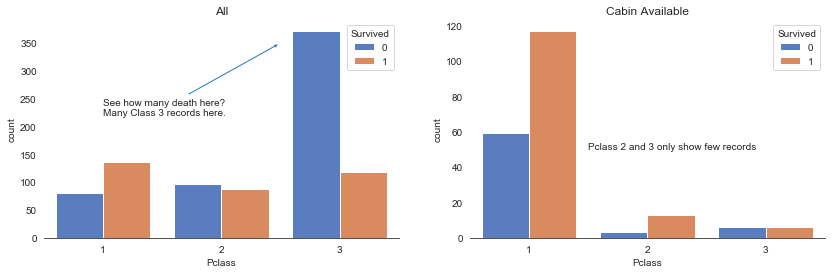Hypothesis proved. I can dig deeper into it but let's now focus on the topic. This column seems to have a high correlation with the fare and pclass and it has many null values. To use that we need more digging and efforts, so we drop that.

In :
df_EDA.drop(['Cabin'], axis=1, inplace=True)

# double check
df_EDA.columns

Out:
Index(['Survived', 'Pclass', 'Name', 'Sex', 'Age', 'SibSp', 'Parch', 'Ticket',
'Fare', 'Embarked', 'Sex_Code'],
dtype='object')

Embarked

We got 2 missing value in the column Embarked. We fill it with the most common value in this column.

In :
print('# of NA in Embarked: ',df_EDA.Embarked.isnull().sum())

df_EDA.Embarked.value_counts()

df_EDA.Embarked.fillna(df_EDA['Embarked'].mode(),inplace=True)

print('Are all the NAs has been filled: ', df_EDA.Embarked.notnull().all())

# of NA in Embarked:  2
Are all the NAs has been filled:  True


### Creating¶

1. Generate title from names.
2. Generate family size and single status from Parch and SibSp.
3. Create bins for fare and age for visualizations
In :
# split the Name column twice to get the title
df_EDA['Title'] = df_EDA.Name.apply(lambda x: x.split(', ').split('. '))

g = sns.countplot(df_EDA['Title'])
g = plt.setp(g.get_xticklabels(), rotation=45)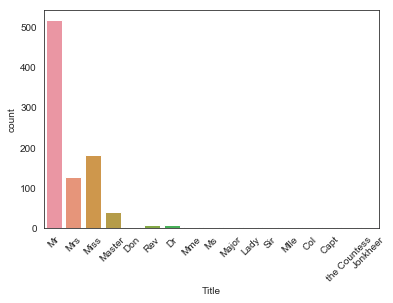In :
# Convert to categorical values Title
df_EDA["Title"] = df_EDA["Title"].replace(['Lady', 'the Countess','Countess','Capt', 'Col','Don', 'Dr', 'Major', 'Rev', 'Sir', 'Jonkheer', 'Dona'], 'Rare')
df_EDA["Title"] = df_EDA["Title"].map({"Master":"Master", "Miss":"Female", "Ms" : "Female" , "Mme":"Female", "Mlle":"Female", "Mrs":"Female", "Mr":"Male", "Rare":"Rare"})

# plot
g = sns.countplot(df_EDA["Title"])
g = g.set_xticklabels(["Master","Miss/Ms/Mme/Mlle/Mrs","Mr","Rare"])
sns.despine()

# create a column of family size and based on it, create another
df_EDA['FamilySize'] = df_EDA['Parch'] + df_EDA['SibSp'] + 1
df_EDA['IsAlone'] = df_EDA['FamilySize'].apply(lambda x: 1 if x == 1 else 0)

# create bins
df_EDA['FareBin'] = pd.qcut(df_EDA['Fare'], 4)
df_EDA['AgeBin'] = pd.cut(df_EDA['Age'].astype(int), 5)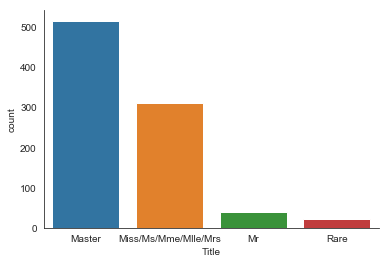### Converting¶

In :
# copy it
df_for_model = df_EDA.copy()

from sklearn.preprocessing import LabelEncoder

# transform the interval/category into code, for EDA purpose
# using pandas astype category because according to Dark417, this performs better in the model
df_EDA['FareBin_Code'] = df_EDA.FareBin.astype('category').cat.codes
df_EDA['AgeBin_Code'] = df_EDA.AgeBin.astype('category').cat.codes
df_EDA['Embarked_Code'] = df_EDA['Embarked'].astype('category').cat.codes


### Train Test Split¶

In :
# converting categorical data into one-hot columns
import patsy as pts

y, X = pts.dmatrices('Survived ~ Pclass + C(Sex) + Age + SibSp + Parch + Fare + ' +
'C(Embarked) + C(Title) + C(Cabin) + FamilySize + IsAlone', data=df_for_model,
return_type='dataframe')
pd.concat([X,y]).info()

from sklearn.model_selection import train_test_split

# train test split
# X_train, X_test, y_train, y_test = \
#     train_test_split(X, y, test_size=0.2, random_state=42)

# I choose to use kfold cv method, so the train test split above is not used anymore
# To keep the rest of the codes unchanged, I'll reassign the variables.

X_train = X
y_train = y

<class 'pandas.core.frame.DataFrame'>
Int64Index: 1782 entries, 0 to 890
Data columns (total 15 columns):
Age                   891 non-null float64
C(Embarked)[T.Q]      891 non-null float64
C(Embarked)[T.S]      891 non-null float64
C(Sex)[T.male]        891 non-null float64
C(Title)[T.Male]      891 non-null float64
C(Title)[T.Master]    891 non-null float64
C(Title)[T.Rare]      891 non-null float64
FamilySize            891 non-null float64
Fare                  891 non-null float64
Intercept             891 non-null float64
IsAlone               891 non-null float64
Parch                 891 non-null float64
Pclass                891 non-null float64
SibSp                 891 non-null float64
Survived              891 non-null float64
dtypes: float64(15)
memory usage: 222.8 KB

In :
# Fare
sns.distplot(df_for_model.Fare)

print("Skewness: {}".format(df_for_model.Fare.skew()))
print("kurtosis: {}".format(df_for_model.Fare.kurtosis()))

Skewness: 4.787316519674893
kurtosis: 33.39814088089868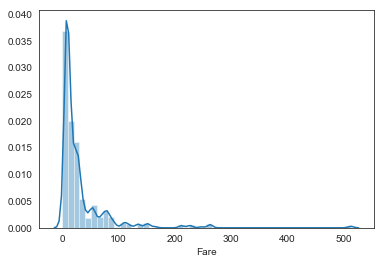In :
df_for_model['Fare'] = df_for_model.Fare.apply(lambda x: np.log(x) if x > 0 else 0)

sns.distplot(df_for_model.Fare)

print("Skewness: {}".format(df_for_model.Fare.skew()))
print("kurtosis: {}".format(df_for_model.Fare.kurtosis()))

Skewness: 0.44310881405404506
kurtosis: 0.641225603709215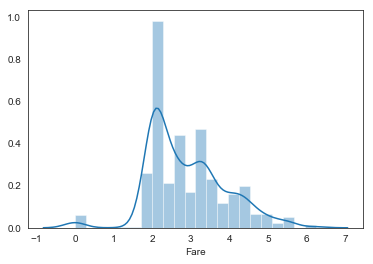## Exploratory Data Analysis¶

Take a quick look of the pairplot and see what we can get.

As pairplot will not show categorical information, we are looking at numerical data.

In :
g = sns.pairplot(df_EDA, hue='Survived', plot_kws={'alpha':0.5,'s':13},
vars=['Sex_Code','Pclass','Fare','Age',\
'FamilySize', 'IsAlone'])
# sns.catplot(kind='')

print(df_EDA.Survived.value_counts(normalize=True).apply(lambda x: '{:.0%}'.format(x)))

0    62%
1    38%
Name: Survived, dtype: object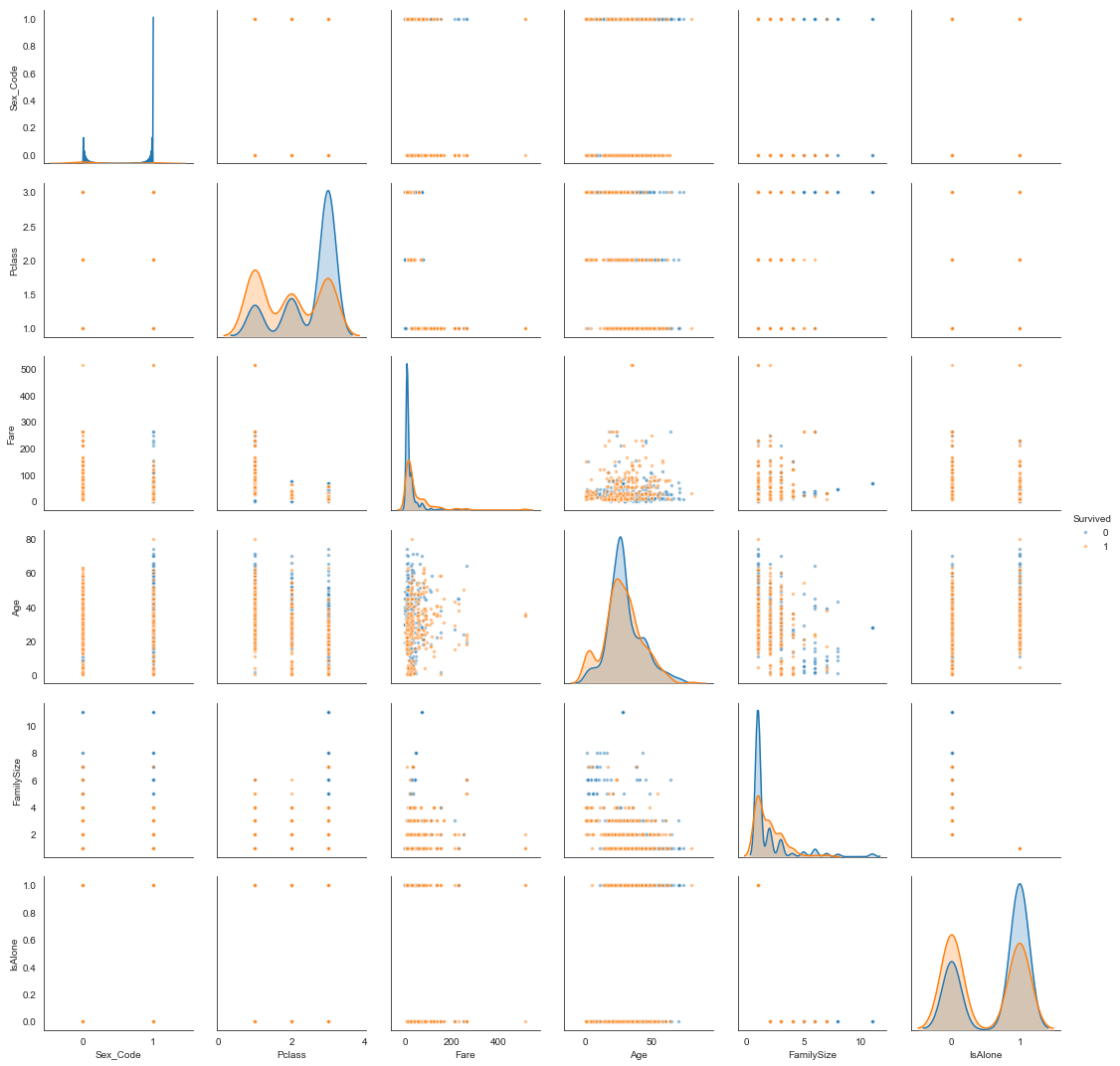Diagonal:

• Fare: a clear peak along the blue line can be seen which means the fatalities are mainly distributed in the low-fare area.
• Age: Children has a higher rate to survive. Mid-age people probably sacrificed themselves?
• Sex: Apparently, women are much more likely to survive than man
• Class: Higher class passenger has a lower rate of death
• Is Alone: stick with family to gain higher survival rate
• Family Size: Don't be alone and don't take too many family friends...

Upper/lower plots: can't see any thing.

Lastly, remember that we have 38% total survival rate.

In :
# I wrote a helper code to display each numeric column as a distplot.
# This is just to understand the distribution of the data.

import helper
tem_df = df_EDA.drop(['Parch', 'SibSp', 'FareBin_Code', 'AgeBin_Code', 'Title'],axis=1)
helper.distplot_w_stats(tem_df)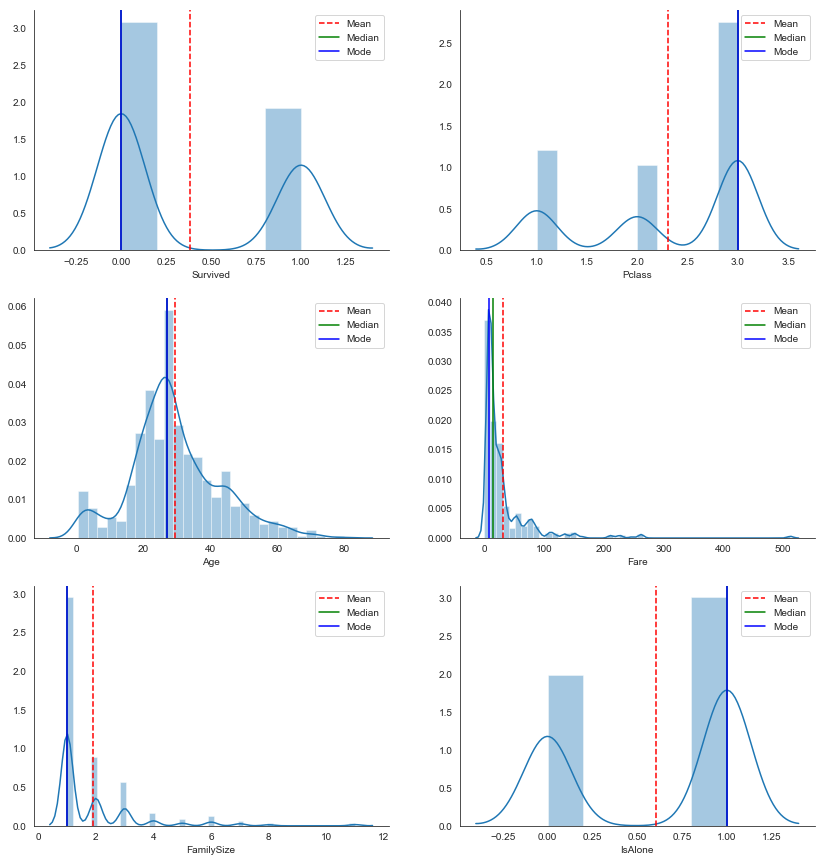In :
tem_df = pd.DataFrame()
tem_df['x'] = pd.cut(df_EDA['Fare'],200).apply(lambda x: round(int(x.mid),0))
tem_df['hue'] = df_EDA['Sex']

# plot
pal = dict(male="#6495ED", female="#F08080")

plt.subplots(figsize=(14,6))
ax = sns.countplot(x='x',hue='hue',data=tem_df,palette=pal)
plt.legend(loc=1)
ax.set_xlim([-.5,30])
ax.set_ylim([1,150])

sns.despine()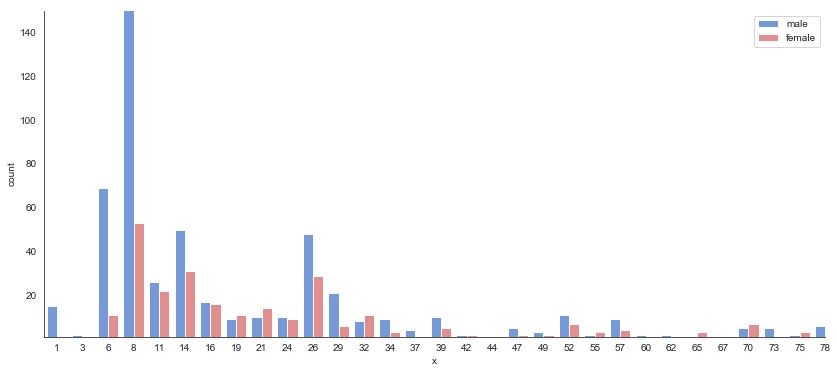### Single and Multi Variable Analysis¶

#### Fare and Pclass¶

In :
sns.set_style('white')

a = sns.jointplot(df_EDA['Fare'], df_EDA['Pclass'],  kind="kde", xlim=(-50,150))

sns.despine()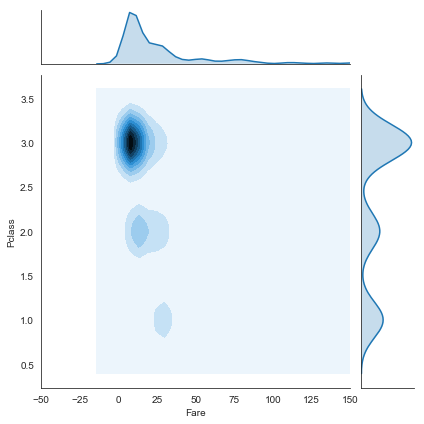In :
pal = dict(male="#6495ED", female="#F08080")

fig, a = plt.subplots(2,3,figsize=(16,9))

ax0 = sns.distplot(df_EDA.Fare, ax=a[0,0])
ax1 = sns.boxplot(df_EDA.Fare, ax=a[0,1],palette="Oranges")
ax2 = sns.barplot(df_EDA['FareBin'].apply(lambda x:int(x.mid)), df_EDA['Survived'], palette='Oranges',ax=a[0,2], alpha=0.5)
sns.lineplot([x for x in range(-1,6)], 0.38, color='red',ax=a[0,2],alpha=.8)

ax0.set_title('Distribution')
ax1.set_title('Distribution')
ax2.set_title('Survival ratio by fare')
ax2.set_xlim(-1,4)
ax2.annotate('38% survival',
(0,.38),
(-.9,.8),
arrowprops=dict(arrowstyle = 'simple'))

a1 = sns.distplot(df_EDA.Pclass,ax=a[1,0])
a2 = sns.countplot('Pclass', hue='Survived', data=df_EDA, palette="BrBG",ax=a[1,1])
a3 = sns.pointplot('Pclass', 'Survived', data=df_EDA, palette=pal,hue='Sex',ax=a[1,2])
sns.lineplot([x for x in range(-1,4)], 0.38, color='red',ax=a[1,2],alpha=.8)

a1.set_title('Distribution')
a2.set_title('Count')
a3.set_title('Survival Ratio')
a3.set_xlim(-1,3)

sns.despine()
print(df_EDA.Fare.describe())

count    891.000000
mean      32.204208
std       49.693429
min        0.000000
25%        7.910400
50%       14.454200
75%       31.000000
max      512.329200
Name: Fare, dtype: float64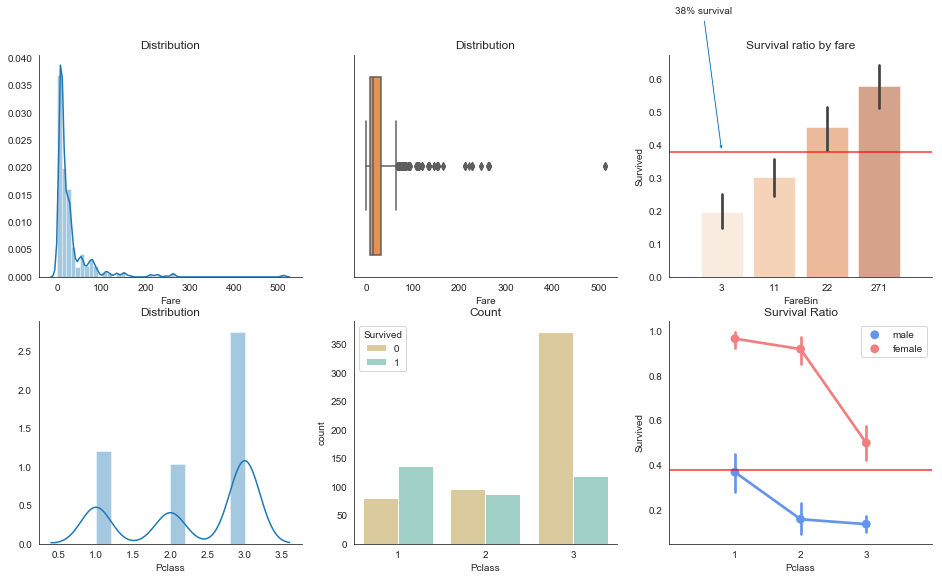Fare Price

1. Distribution Plot:

Most people paid 7.91 ~ 31.00 for the ticket, while some paid a lot of money like 512.32... We can see there are some outliers in the box plot. From the stats data showed above, we know that the mean value is influenced by the outliers, and the std is quite high possibly for the same reason.

1. Line and Bar Plot:

In the graph, we have 4 groups of bins, and each of them has exactly the same number as each other because we cut the fare using Quantile-based discretization function.

As expected, we found that the more money paid for the ticket, the higher survival rate it will be.

Pclass

1. Most people are in class 3
2. Only class 1 has the survival rate > 50%
3. Class 3 has a low survival rate

Expected. Same reason as fare price.

Sex

1. Women > men.
2. Even a first class man, his survival rate < 38%.

Gentle man. My greatest respect.

#### Four Dimensional Analysis¶

Variables: Age, Pclass, Survived, Sex

In :
pal = dict(male="#6495ED", female="#F08080")

# Show the survival proability as a function of age and sex
g = sns.lmplot(x="Age", y="Survived", row = 'Pclass', col="Sex", hue="Sex", data=df_EDA, aspect=2,
palette=pal, y_jitter=.02, logistic=True)

g.set(xlim=(0, 80), ylim=(-.05, 1.05))

Out:
<seaborn.axisgrid.FacetGrid at 0x133384b1400>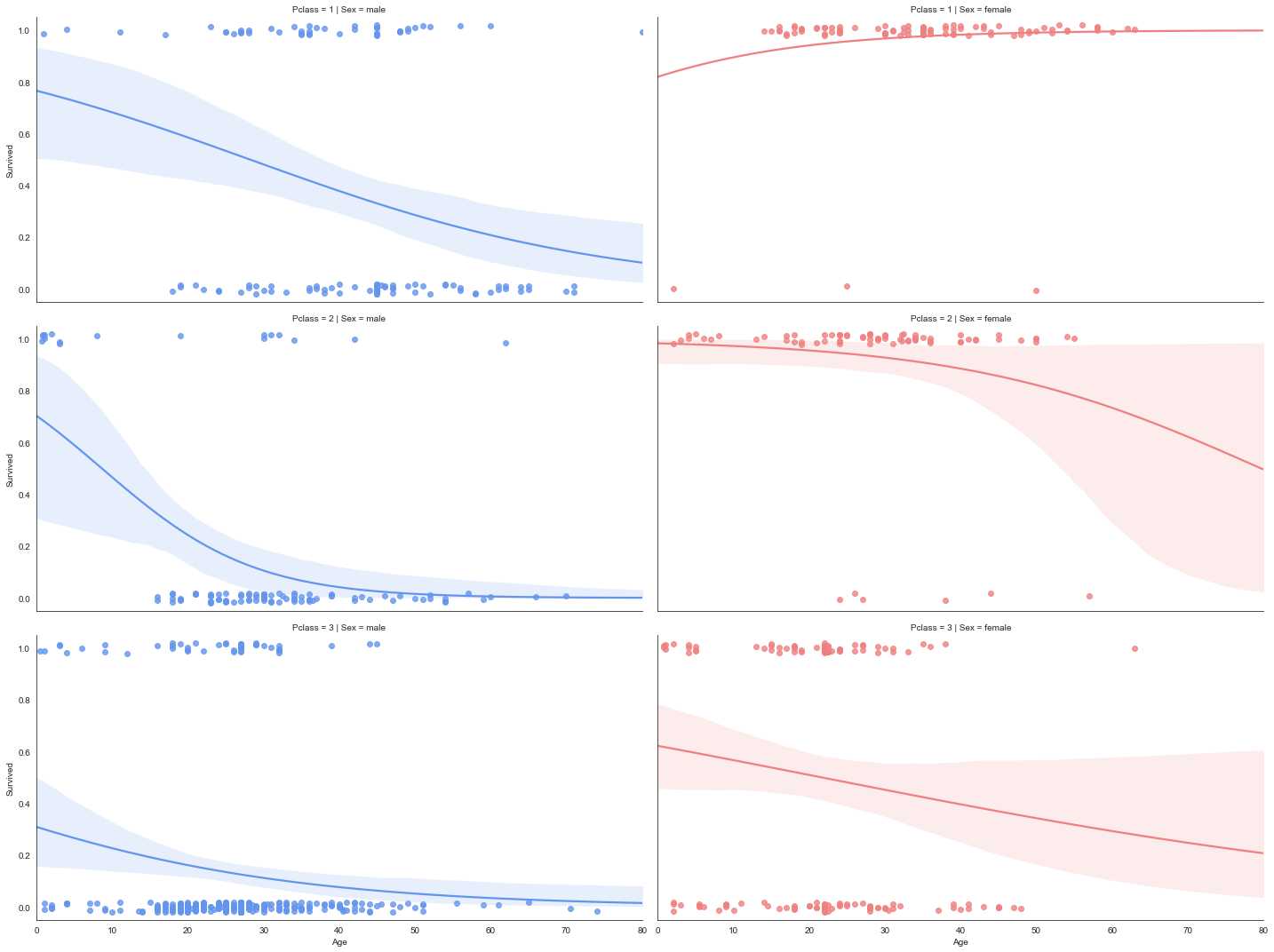Regression Plot

• Most ladies in class one were saved, the survival rate them is extremely high
• Older ladies in class 2 have a chance to be abandoned
• Boys in Class 2 have a high rate of survival, maybe class 2 people gives their lives for the young ones.
• As the age increases, the rate of survival decreases (for most cases)
• Class 1 > class 2 > class 3, class 3 sucks
• From the left three charts, we can tell that there has a clear linear relationship between age and survived.
In :
g = sns.factorplot(x="Pclass", y="Age", hue="Survived", col="Sex",
data=df_EDA, kind="violin", split=True,
bw=0.05, size=7, aspect=.9, s=7)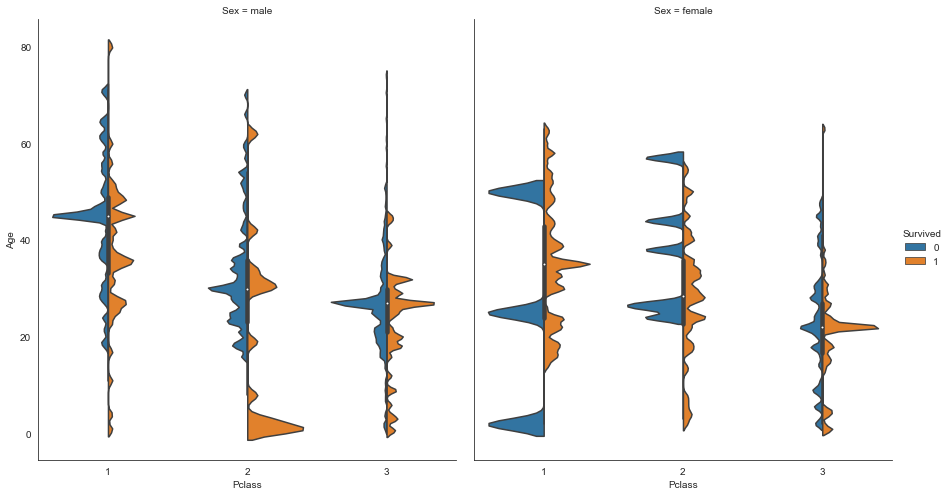Violin plots

This is another way to display a 4 dimensional chart. While the lmplots emphases on the relationships between x and y, violin plots focuses on showing the distribution of the survivors.

• Class 2 boys are all saved!
• A peak in female class 3: it shows that most of the ladies on in this age.
• Some peaks can be misleading because of lack of data... e.g. female, pclass 1 and 2, not survived. However, if you know the dataset well enough, you will know there are a few females unfortunately die in this event from class 1 and 2 and these peaks demonstrate that.
• From the tail/head of the distribution, we can see that there are more old men than old women

#### Ticket¶

This is a tricky one and I don't wanna go too deep on that, but let's try it anyway. From the numbers, we can know the sequence of booking and something more, but I really don't think this will contribute much on the analysis.

Instead, let's see what's the survival rate for different strings included in the ticket.

In :
# replacing all digit with empty strings, and show the result
def replace_digit(x):
result = ""
for i in x:
if not i.isdigit():
result = "".join([result,i])
return result.strip()

# save the result in a variable
converted_ticket = train_df['Ticket'].apply(replace_digit)

# create a slicer including top 5 values of the above variable
slicer = train_df['Ticket'].apply(replace_digit).value_counts().index[:5]

In :
j = []
k = []

for i in slicer:
print('Testing for {}'.format(i))
print('Number of data: {}'.format(sum(converted_ticket == i)))
j.append(i)
try:
survival_rate = train_df[converted_ticket == i].Survived.value_counts(normalize=True).loc
print('P(Survival) = {:.0%}'.format(survival_rate))
k.append(survival_rate)

except:
print('The rate of survival is 0')
k.append(0)

print('-'*20)

f, axis = plt.subplots(figsize=(10,4))
a1 = sns.barplot(j,k,palette='GnBu_d')
sns.lineplot(range(-1,len(j) + 1),[.38 for j in range(len(j) + 2)],color='red')
a1.set_xlabel('String in Ticket')
a1.set_ylabel('Survival Rate')
a1.set_xlim(-1,5)

sns.despine()

Testing for
Number of data: 661
P(Survival) = 38%
--------------------
Testing for PC
Number of data: 60
P(Survival) = 65%
--------------------
Testing for C.A.
Number of data: 27
P(Survival) = 48%
--------------------
Testing for A/
Number of data: 13
P(Survival) = 8%
--------------------
Testing for STON/O .
Number of data: 12
P(Survival) = 42%
--------------------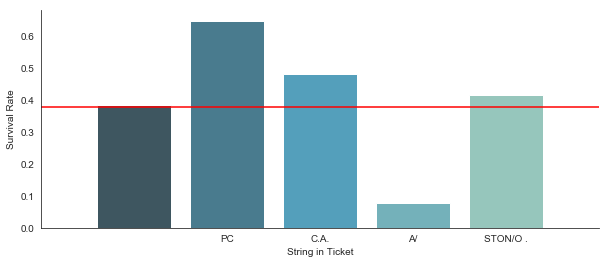As we already know that the rate of survival for the entire training set is 38%, the first result proves that again. The second result shows a 65% of survival rate. Is that merely a coincidence? Does PC stands for premium class? We should see correlations between different columns later.

The rest of the results cannot prove anything due to lack of data.

In :
# we convert the ticket to another column
# will use it later
converted_ticket = converted_ticket.apply(lambda x: 1 if x == 'PC ' else 0)


#### Cabin¶

In :
Cabin_transformed = train_df.Cabin.apply(lambda x: replace_digit(str(x)))
Cabin_counts = train_df.Cabin.apply(lambda x: replace_digit(str(x))).value_counts()
Cabin_filter = Cabin_counts > 10

Cabin_transformed = Cabin_transformed.fillna('nan').apply(lambda x: x if Cabin_filter[x] else 'misc')

In :
a = sns.barplot(Cabin_transformed,train_df.Survived, palette='Blues')
sns.lineplot(range(-1,8),[.38 for x in range(9)],color='red')

a.set_xlim(-1,7)
sns.despine()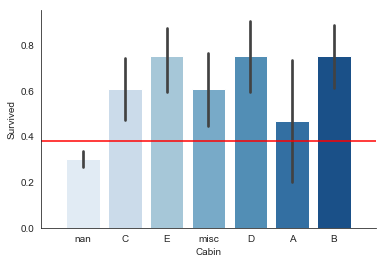Remember, we only have 200+ number of data in Cabin, which only takes 25% of the total.

Very interestingly, We can see the survival rates for Cabin with letters in them all exceed the overall fatality rate. The rest of records that have no Cabin data with them have a lower than overall survival rate - 30%.

#### Embarked¶

In :
print('*'*35)
print('Survival Rate wrt Embarked')
print(df_EDA.pivot_table(values='Survived',columns=['Embarked']))
print('*'*35)

print('*'*35)
print('Embarked Count')
print(df_EDA.Embarked.value_counts())
print('*'*35)
g = sns.FacetGrid(df_EDA, col = 'Embarked', height =4)
g.map(sns.pointplot,'FareBin', 'Survived', 'Sex', palette='muted')

sns.despine()

***********************************
Survival Rate wrt Embarked
Embarked         C        Q         S
Survived  0.553571  0.38961  0.339009
***********************************
***********************************
Embarked Count
S    646
C    168
Q     77
Name: Embarked, dtype: int64
***********************************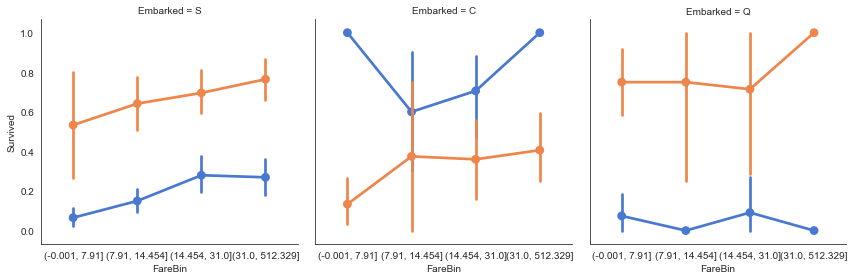• People embarked at C has a higher rate of survival. Maybe they have higher class ticket?
• Men's survival rate is higher than women in C.

### Correlations between data¶

• Chart 1: Feature Correlation w.r.t. Survival
• Chart 2: Correlations between all features
In :
corr_data = (df_EDA.corr()
.drop(['Survived'])['Survived']
.apply(abs)
.sort_values(ascending=True)
)

(corr_data
.apply(lambda x: '{:.2%}'.format(x))
.to_frame('Correlation with Survival')
)

Out:
Correlation with Survival
FamilySize 1.66%
AgeBin_Code 3.49%
SibSp 3.53%
Age 6.52%
Parch 8.16%
Embarked_Code 16.77%
IsAlone 20.34%
Fare 25.73%
FareBin_Code 29.94%
Pclass 33.85%
Sex_Code 54.34%
In :
sns.set(style = 'white')
plt.subplots(figsize=(12,4))

ax = sns.barplot(corr_data.values,
corr_data.index,
orient='h',
palette='RdBu')

ax.set_title('Featrue Correlation with Survival')

sns.despine()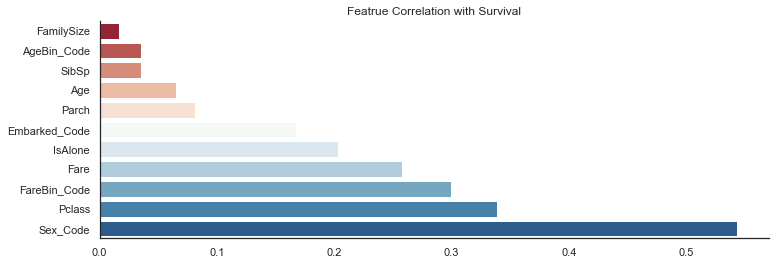• Sex has the largest correlation value with survival. We've seen the difference in EDA. Expected.
• Ticket class and Fare follows. We have fare, fare bins and pclass, and all of them are social class indicators. We should the correlations between these three features.
• Is alone: this is a great column created from Parch + SibSp. Nice job.
• Embarked: we've know that people embarked at C have greater chance to survive.
In :
sns.set(style = 'darkgrid')

cmap = sns.diverging_palette(220, 10, as_cmap=True)

f, ax = plt.subplots(figsize=(11, 9))

sns.heatmap(df_EDA.corr(),
center=0,
cmap = cmap,
square=True,
ax=ax,
cbar_kws=